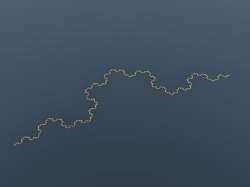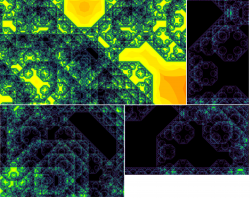News: Follow us on Twitter## The All New FractalForums is now in Public Beta Testing! Visit FractalForums.org and check it out!

On the CurvePrevious Image | Next Image
Description: If anyone is interested I have been trying to clean out my 2016 backlog of experiments and images onto my facebook page so if you use it then feel free to check out the heaps of stuff on there and as ever ask for any params you like the look of . www.facebook.com/timemit-393093930773750

// Output generated from file: C:/Users/USER/Desktop/Fragmentarium/Examples/Knighty Collection/PseudoKleinian_4D_Quaternion_Julia.frag
// Created: Wed Nov 23 19:51:21 2016
#info Knighty's Pseudo Kleinian (Scale 1 JuliaBox + Something)
#info Modified by Crist-JRoger 4D Quaternion Julia
#info http://www.fractalforums.com/fragmentarium/fragmentarium-an-ide-for-exploring-3d-fractals-and-other-systems-on-the-gpu/msg81393/#msg81393
#include "MathUtils.frag"
#include "DE-Kn2cr11.frag"

#group 4D Quaternion Julia

uniform int jIterations;  slider[0,16,100]
uniform float jThreshold; slider[0,10,100]
uniform vec4 jC; slider[(-1,-1,-1,-1),(0.18,0.88,0.24,0.16),(1,1,1,1)]
float DE1(vec3 pos) {
vec4 p = vec4(pos, 0.0);
vec4 dp = vec4(1.0, 0.0,0.0,0.0);
for (int i = 0; i < jIterations; i++) {
dp = 2.0* vec4(p.x*dp.x-dot(p.yzw, dp.yzw), p.x*dp.yzw+dp.x*p.yzw+cross(p.yzw, dp.yzw));
p = vec4(p.x*p.x-dot(p.yzw, p.yzw), vec3(2.0*p.x*p.yzw)) + jC;
float p2 = dot(p,p);
orbitTrap = min(orbitTrap, abs(vec4(p.xyz,p2)));
if (p2 > jThreshold) break;
}
float r = length(p);
return  0.5 * r * log(r) / length(dp);
}

#group PseudoKleinian

#define USE_INF_NORM

uniform int MI; slider[0,25,100]

// Bailout
//uniform float Bailout; slider[0,20,1000]

uniform float Size; slider[0,1,4]
uniform vec3 CSize; slider[(0,0,0),(1,1,1),(4,4,4)]
uniform vec3 C; slider[(-4,-4,-4),(0,0,0),(4,4,4)]
uniform float TThickness; slider[0,0.01,2]
uniform float DEoffset; slider[0,0,0.01]
uniform vec3 Offset; slider[(-1,-1,-1),(0,0,0),(1,1,1)]

float RoundBox(vec3 p, vec3 csize, float offset)
{
vec3 di = abs(p) - csize;
float k=max(di.x,max(di.y,di.z));
return abs(k*float(k<0.)+ length(max(di,0.0))-offset);
}

float maxcomp(vec3 a) {
return     max(a.x,max(a.y,a.z));
}

float sdToBox( vec3 p, vec3 b )
{
vec3  di = abs(p) - b;
float mc = maxcomp(di);
return min(mc,length(max(di,0.0)));
}

float Thingy(vec3 p, float e){
p-=Offset;
return (abs(length(p.xy)*p.z)-e) / sqrt(dot(p,p)+abs(e));
}

float Thing2(vec3 p){
//Just scale=1 Julia box
float DEfactor=1.;
vec3 ap=p+1.;
for(int i=0;i<MI && ap!=p;i++){
ap=p;
p=2.*clamp(p, -CSize, CSize)-p;

float r2=dot(p,p);
orbitTrap = min(orbitTrap, abs(vec4(p,r2)));
float k=max(Size/r2,1.);

p*=k;DEfactor*=k;

p+=C;
orbitTrap = min(orbitTrap, abs(vec4(p,dot(p,p))));
}
//Call basic shape and scale its DE
//return abs(0.5*Thingy(p,TThickness)/DEfactor-DEoffset);

//Alternative shape
//return abs(0.5*RoundBox(p, vec3(1.,1.,1.), 1.0)/DEfactor-DEoffset);
//Just a plane
return abs(0.5*abs((p.z-Offset.z)*DE1(p))/DEfactor-DEoffset);
}

float DE(vec3 p){
return  Thing2(p);//RoundBox(p, CSize, Offset);
}

#preset OntheCurve
FOV = 0.7201087
Eye = -2.556512,-18.73893,-8.636192
Target = -3.839587,-16.37893,-14.67872
Up = -0.2597066,0.245291,0.1509486
Gamma = 0.8654
ToneMapping = 5
Exposure = 1.344033
Brightness = 1
Contrast = 1
Saturation = 1
GaussianWeight = 1
AntiAliasScale = 2
Detail = -3.68141
FudgeFactor = 0.4297812
Dither = 0.0469289
NormalBackStep = 4.379928 NotLocked
CamLight = 0.760784,0.870588,1,0.0282486
BaseColor = 1,1,1
OrbitStrength = 0.9817672
X = 0.5,0.6,0.6,0.4896552
Y = 1,0.6,0,0.497931
Z = 0.8,0.78,1,0.7806897
R = 0.4,0.7,1,0.2822636
BackgroundColor = 0.7803922,0.5568627,0.2980392
CycleColors = true
Cycles = 5.057251
EnableFloor = false NotLocked
FloorNormal = 0,0,1
FloorHeight = 0
FloorColor = 1,1,1
FocalPlane = 8.875221
Aperture = 0
InFocusAWidth = 0.9629893
ApertureNbrSides = 7
ApertureRot = 0
ApStarShaped = false
Bloom = false
BloomIntensity = 0.7761194
BloomPow = 1.425562
MaxRaySteps = 2000
MaxDistance = 20
Specular = 0.1626465
SpecularExp = 16
Reflection = 0.7803922,0.9803922,1
ReflectionsNumber = 2
SpotGlow = true
SpotLight = 1,0.8313725,0.4941176,5.756272
LightPos = 2.030812,-0.1960784,1.162465
LightSize = 0.0800836
LightFallOff = 0
LightGlowExp = 1
HF_Fallof = 0.0696016
HF_Const = 0.0145329
HF_Intensity = 0.0412587
HF_Dir = 0,-0.4010989,1
HF_Offset = 3.170391
HF_Color = 0.7960784,1,0.9960784,1.220339
HF_Scatter = 0
HF_Anisotropy = 0,0,0
HF_FogIter = 1
CloudScale = 1
CloudTops = 1
CloudBase = -1
CloudDensity = 1
CloudRoughness = 1
CloudContrast = 1
CloudColor = 0.65,0.68,0.7
SunLightColor = 0.7,0.5,0.3
UpLock = false
DofCorrect = false
BloomTaps = 19
BloomStrong = 5.37323
RefineSteps = 15
DetailAO = -0.0532503
coneApertureAO = 0.5
maxIterAO = 20
FudgeAO = 1
AO_ambient = 1.979065
AO_camlight = 1.76257
AO_pointlight = 1.964962
AoCorrect = 0.8745669
AmbiantLight = 1,1,1,0.4592275
perf = false
SSS = true
sss1 = 0.4516129
sss2 = 0.5
EnCloudsDir = false
Clouds_Dir = 0,0,1
CloudFlatness = 0
CloudColor2 = 0.07,0.17,0.24
Cloudvar1 = 0.99
Cloudvar2 = 1
CloudIter = 5
CloudBgMix = 1
jIterations = 2
jThreshold = 9.72028
jC = -0.8064953,0.4465494,-0.4221922,-0.3585927
MI = 99
Size = 0.4453496
CSize = 0.3765348,0.4448175,0.3627622
C = -0.4942606,1.088454,-1.671843
TThickness = 0
DEoffset = 0
Offset = -0.5374056,-1,-0.3919012
#endpreset

Stats:
Total Favorities: 0 View Who Favorited
Filesize: 662.42kB
Height: 1080 Width: 1920
Discussion Topic: View Topic
Keywords: Fragmentarium..timemit Knighty. Psuedokleinian quaternion
Posted by: Tim EmitDecember 08, 2016, 08:09:04 PM

Rating: Has not been rated yet.

 Dragon CurveRating: (None)Filesize: 73.48kBDate: October 11, 2009, 08:18:07 PMComments (0)By: Guest Lévy C CurveRating:Filesize: 83.14kBDate: October 11, 2009, 08:18:08 PMComments (0)By: Guest Koch-curveRating:Filesize: 47.18kBDate: May 16, 2010, 10:33:27 PMComments (0)By: knighty The C curveRating:Filesize: 7.07MBDate: February 14, 2016, 01:04:51 AMComments (0)By: Fractal universe Details of the C curveRating: (None)Filesize: 12.14MBDate: February 15, 2016, 02:12:39 PMComments (0)By: Fractal universe